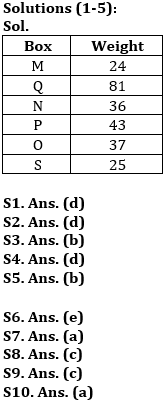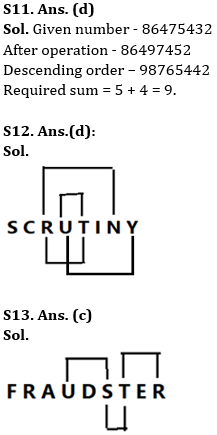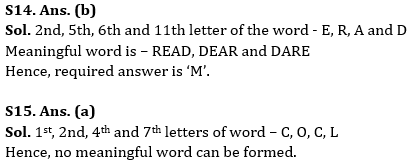Latest Banking jobs   »

# Reasoning Ability Quiz For Bank Foundation 2023 -10th April

Six boxes M, N, O, P, Q, and S are placed one above another, also they are of different weights (in kg.) i.e., 24, 25, 36, 37, 43, and 81(but not necessarily in the same order). Box P’s weight is not a lowest among all the boxes. M is placed either top or bottom. The weight of the box which is placed at bottom is 25kg. Only one box is placed between P and S. Box Q is not placed just above and below P. Box O is placed below Q but not just below Q. The weight of the box which is placed just above N is a perfect square of an odd number. Box O’s weight is a prime number. Box N is not placed above M whose weight is not a perfect square. Only 2 boxes are placed between M and P whose weight is a prime number. Box P is not third heaviest box.

Q1. What is the weight of Box O?
(a) 24
(b) 25
(c) 36
(d) 37
(e) None of these

Q2. Which of the following box is placed at top?
(a) N
(b) S
(c) O
(d) M
(e) None of these

Q3. How many boxes are placed between Q and the box which weight’s is 25?
(a) One
(b) Three
(c) Two
(d) More than three
(e) None of these

Q4. Which of following box is 24kg?
(a) P
(b) N
(c) Q
(d) M
(e) None of these

Q5. What is the weight of the box which is placed immediate below N?
(a) 36
(b) 43
(c) 24
(d) 81
(e) None of these

Directions (6-10): Study the following arrangement to answer the given questions

U 3 K % S I \$ V 8 E 5 G © O 4 P @ B 7 Z # 6 & N * 9 R A 4 1 X

Q6. Which of the following element is third to the left of the ninth to the right of ‘O’?
(a) 6
(b) #
(c) 9
(d) Q
(e) None of these.

Q7. Which of the following element is the eighth to the right of the fourteenth from the left end of the above arrangement?
(a) 6.
(b) #
(c) 9
(d) Q
(e) None of these

Q8. How many such numbers are there in the above arrangement, each of which is immediately preceded by a vowel and immediately followed by a consonant?
(a) One
(b) Two
(c) Three.
(d) More than four
(e) None

Q9. What should come next in the following series based on the above arrangement?
(a) U3K
(b) 6*A
(c) 6*R.
(d) 691
(e) &6N

Q10. If we insert “T” after every third letter in the above series starting from the left end of the series, then what will be the 19th element from the right end of the series?
(a) B.
(b) T
(c) @
(d) 7
(e) None of these

Q11. If all the odd digits of the number “86475432” are increased by ‘2’ and then all the digits are arranged in descending order from left to right, then what will be the sum of fifth digit from left end and second digit from the right end?
(a) 12
(b) 10
(c) 8
(d) 9
(e) None of these

Q12. How many pairs of letters are there in the word “SCRUTINY” which has as many letters between them in the word as in the alphabetical series (Both forward and backward direction)?
(a) One
(b) Two
(c) Three
(d) Four
(e) More than four

Q13. How many pairs of letters are there in the word “FRAUDSTER” which has as many letters between them in the word as in the alphabetical series (Both forward and backward direction)?
(a) One
(b) Two
(c) Three
(d) Four
(e) More than four

Q14. If it is possible to make a meaningful word using the second, fifth, sixth and eleventh letters of the word ‘CENTRALISED’, then what is the fourth letter of the word formed from left end? If no such word can be formed, then mark the answer as ‘T’. If more than one word can be formed, then mark the answer as ‘M’.
(a) T
(b) M
(c) D
(d) A
(e) R

Q15. If it is possible to make a meaningful word from 1st, 2nd, 4th and 7th letters of word “CONCEALMENT”, then which will be the 2nd letter of the word from left end? If no such word can be formed, then mark the answer as ‘N’. If more than one word can be formed mark the answer as ‘M’.
(a) N
(b) M
(c) C
(d) O
(e) L

Solutions## FAQs

### What is the selection process of the Bank Clerk?

The selection process of the Bank Clerk is Prelims & Mains.

#### Congratulations!Union Budget 2023-24: Free PDF